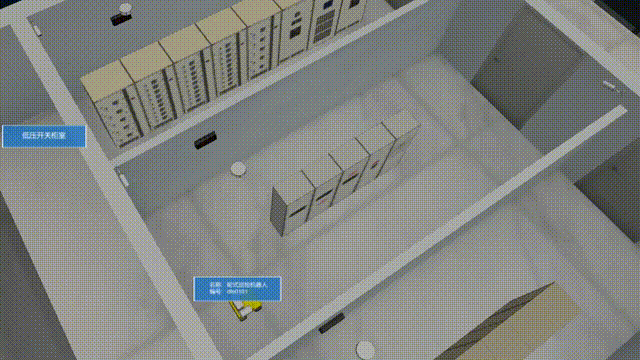# 三维场景中常用的路径动画

## 直线路径动画

``````const points = [A, B];
// 创建路径
let path = new mono.Path();
points.forEach((point, i) => {
const { x, y, z } = point;
if (i == 0) {
path.moveTo(x, y, z);
} else {
path.lineTo(x, y, z);
}
});
// 动画
const instance = new mono.Animate({
form: 0,
to：1,
dur：3000,
delay,
reverse：false,
repeat: 1,
easing,
onPlay,
onUpdate: (val) => {
// 获取路径上的点
const point = path.getPointAt(val);
// 设置实体位置
entity.setPosition(point);
},
onDone,
});
instance.play();

## 折线路径动画

``````const points = [A, B, C, D];
// 创建路径
let path = new mono.Path();
points.forEach(...);
// 动画
const instance = new mono.Animate({
...
onUpdate: (val) => {
// 获取路径上的点
const point = path.getPointAt(val);
// 设置实体位置
entity.setPosition(point);
},
onDone,
});
instance.play();

### 折线路径闭环动画

``````const points = [A, B, C, D];
// 创建路径
let path = new mono.Path();
points.forEach(...);
// 闭环路径
path.closePath()
// 动画
const instance = new mono.Animate({
...
});
instance.play();

### 圆润的折线路径动画

``````const points = [A, B, C, D];
// 创建路径
let path = new mono.Path();
points.forEach(...);
// 闭环路径
path.closePath()
// 获取平滑路径, 让转弯更自然
// 动画
const instance = new mono.Animate({
...
});
instance.play();

### 模型与路径动画同步旋转

``````const points = [A, B, C, D];
// 创建路径
let path = new mono.Path();
points.forEach(...);
// 闭环路径 获取平滑路径, 让转弯更自然
...
// 旋转向量
const rotate = new mono.Vec3();
// 动画
const instance = new mono.Animate({
onUpdate: (val) => {
// 位置
...
// 模型同步旋转
const tangent = path.getTangentAt(val);
var normal = new mono.Vec3(0, 0, -1);
rotate.rotationTowards(normal, tangent);
entity.setRotation(rotate);
}
});
instance.play();那么，直线路径动画和折线路径动画介绍完了。从上面动画截图中可以看出，我们是在一个固定的位置查看动画，那么，能让镜头沿着路径一起移动么

### 镜头沿路径动画一起移动

``````const points = [....];
// 创建路径
let path = new mono.Path();
points.forEach(...);
// 闭环路径 获取平滑路径, 让转弯更自然
...
// 镜头初始位置
const pos = camera.p(),
target = camera.t();
const length = pos.clone().sub(target).length();
// 动画
const instance = new mono.Animate({
onUpdate: (val) => {
// 模型
...
// 镜头沿路径动画
const tangent = path.getTangentAt(value);
point = path.getPointAt(value);
camera.p(point);
camera.lookAt(ntarget);
}
});
instance.play();

### 镜头与路径保持平行一起移动

``````const points = [....];
// 创建路径
let path = new mono.Path();
points.forEach(...);
// 闭环路径 获取平滑路径, 让转弯更自然
...
// 镜头相对于目标动画模型的距离
const dis = 550
// 动画
const instance = new mono.Animate({
onUpdate: (val) => {
// 模型
...
// 镜头平行X轴动画
point = path.getPointAt(value);
camera.lookAt(point);
}
});
instance.play();